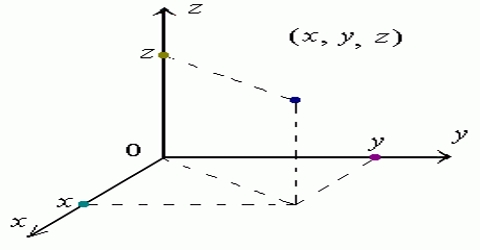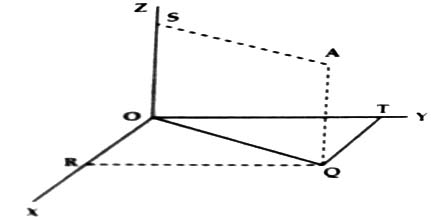Physics

# Three Dimensional Reference FrameThree dimensional reference frame:

Let a particle be situated in a room full of air. In order to locate the position of the particle three mutually perpendicular straight lines are needed. Let the three straight lines be respectively OX, OY and OZ. These three lines have intersected at point O. So, O is the origin or reference point. Here OX is called X-axis; OY, the Y-axis and OZ, the Z-axis. The frame which has been formed by the origin O and the three axes is called three dimensional reference frame [Figure].Let the particle be at position A at a particular time. Let us draw a normal AQ from A on the plane XY. Similarly, normal QR is drawn on OX and normal QT is drawn on OY from the point Q. Hence,

OR = QT = x

OT = RQ = y

and OS = AQ = z.

Here the co-ordinates of A has been designated by co-ordinates x, y and z. The frame containing the origin O and the three co-ordinates is called the three dimensional frame.

If different particles of a body are located in this frame, then the body is called three dimensional body.

Example: Table, chair, brick, stone etc. are three dimensional objects.